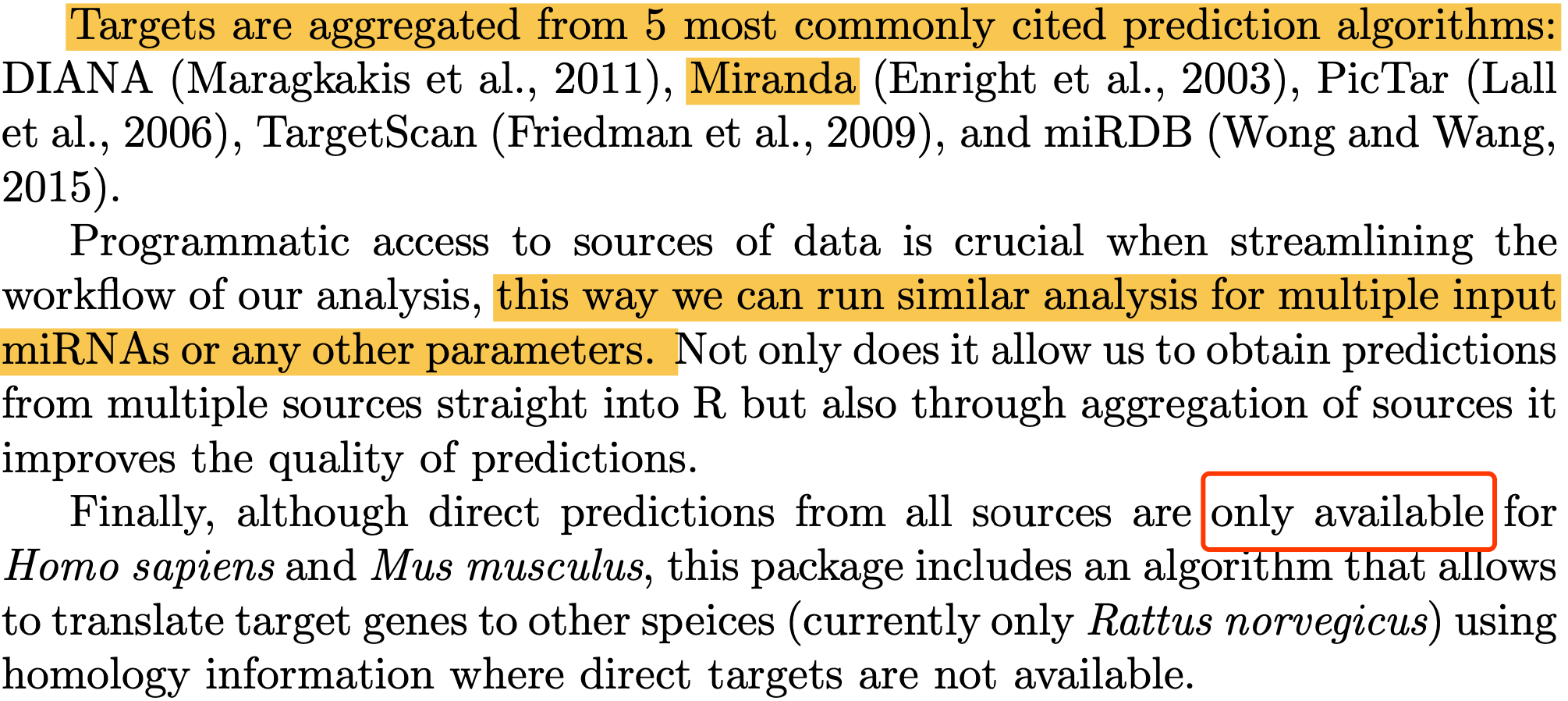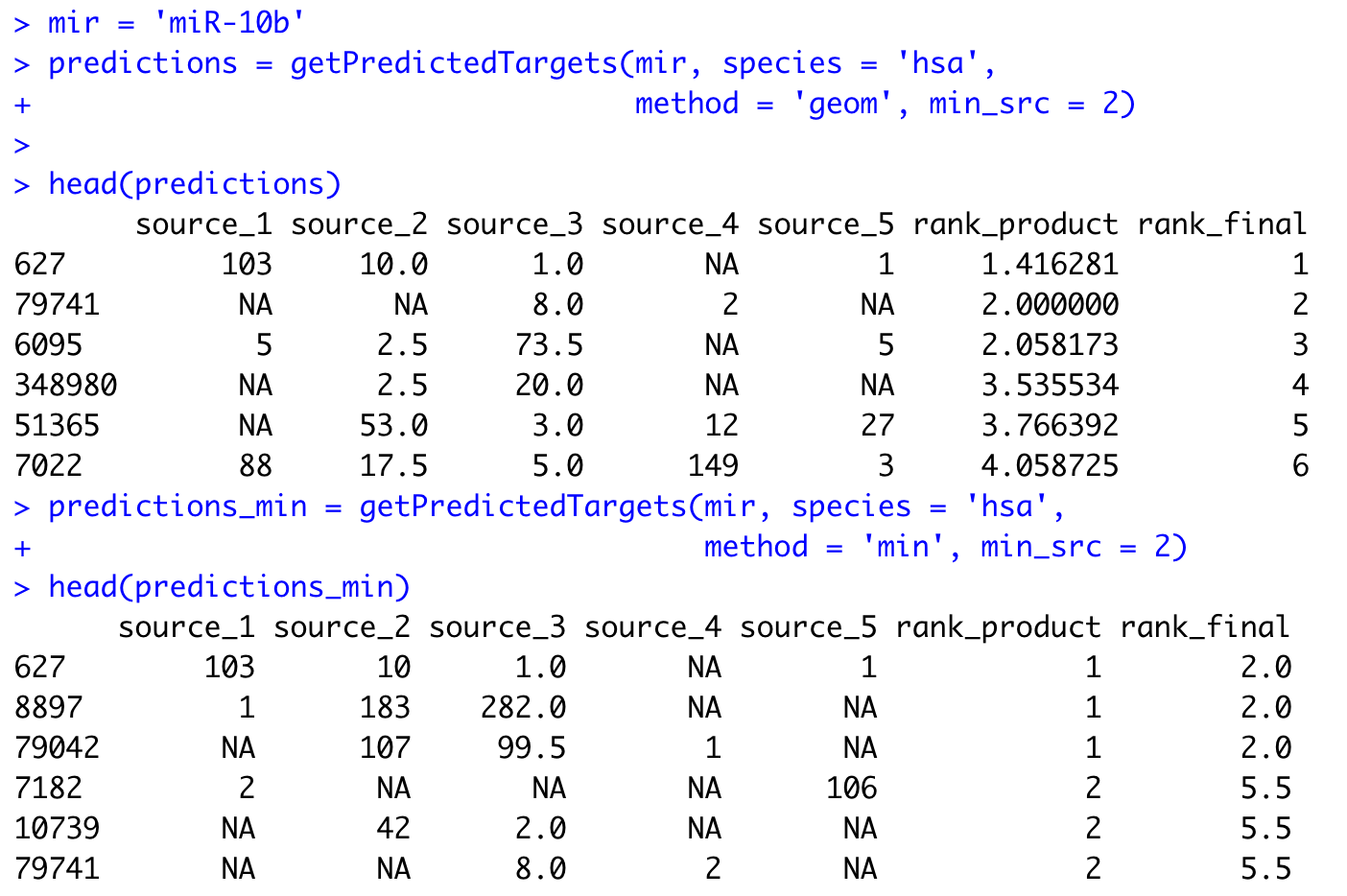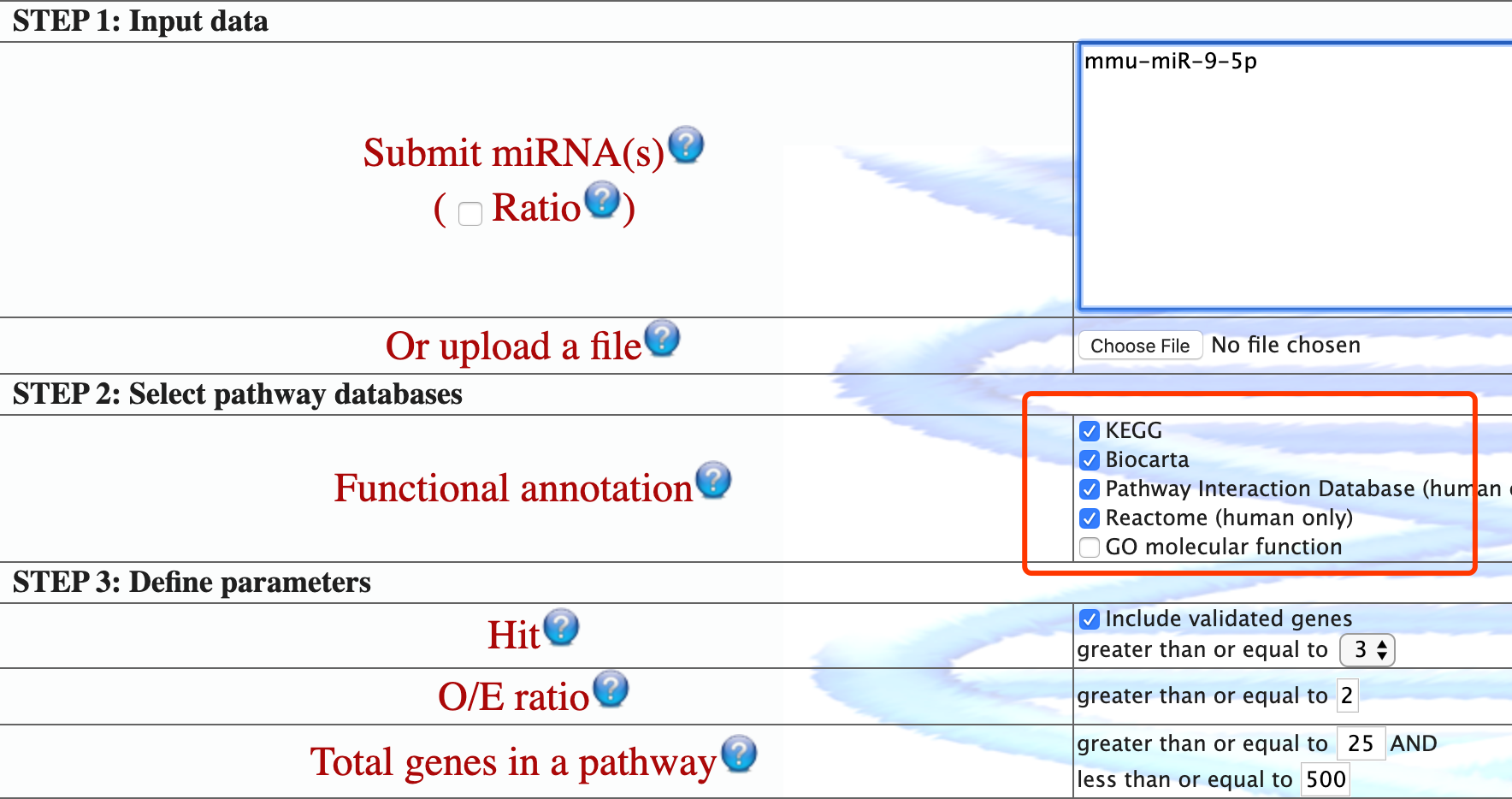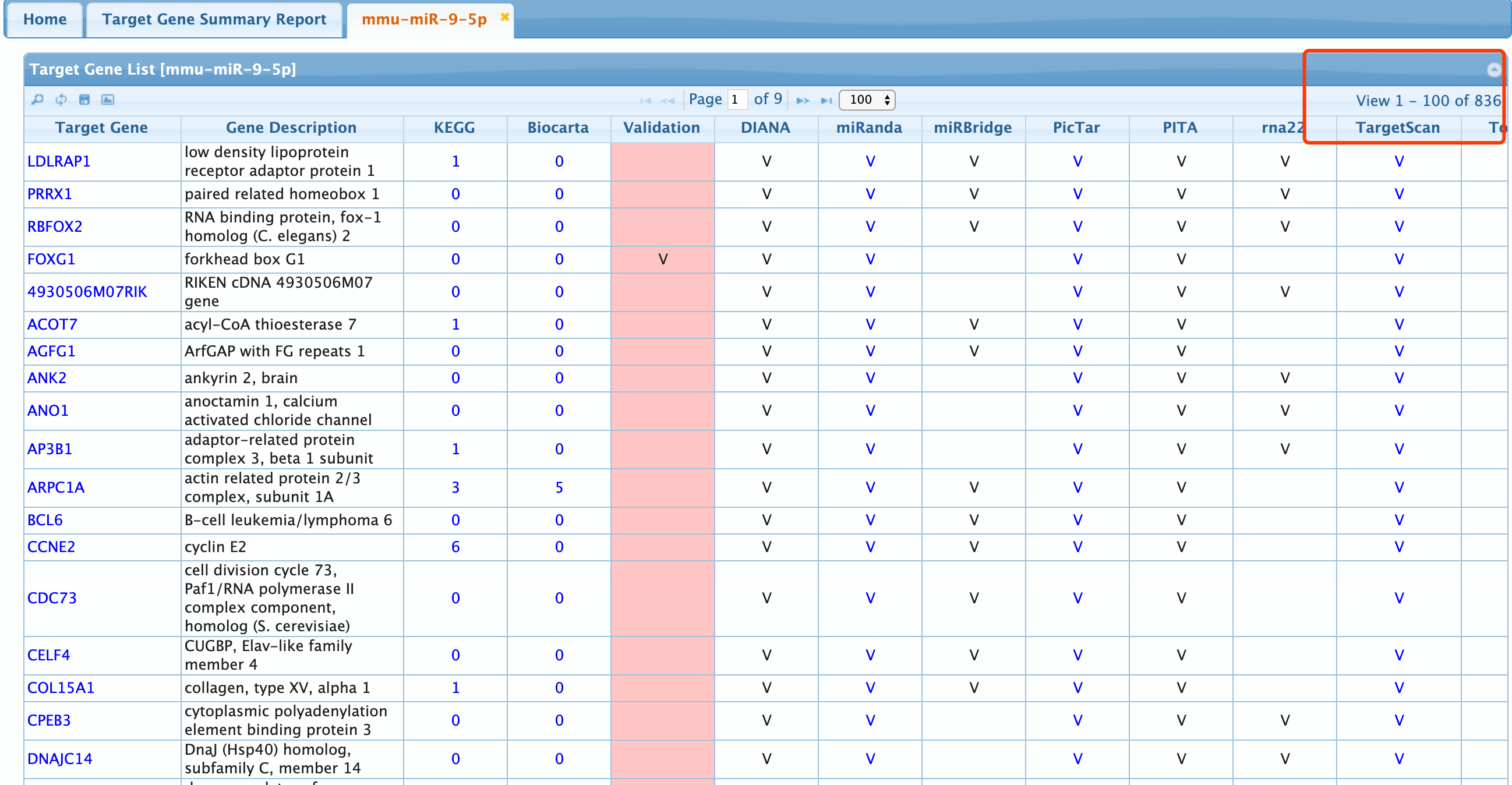# 使用miRNAtap数据源提取miRNA的预测靶基因结果

``````options(BioC_mirror="https://mirrors.tuna.tsinghua.edu.cn/bioconductor/")
options("repos" = c(CRAN="http://mirrors.cloud.tencent.com/CRAN/"))
options(url.method='libcurl')

````````````rm(list = ls())
library(miRNAtap)
library(topGO)
library(org.Hs.eg.db)
mir = 'miR-10b'
predictions = getPredictedTargets(mir, species = 'hsa',
method = 'geom', min_src = 2)

predictions_min = getPredictedTargets(mir, species = 'hsa',
method = 'min', min_src = 2)
```````min'` is a minimum of ranks, `'max'` is a maximum of ranks, and default `'geom'`is based on geometric mean of the ranks which proves to be the most accurate method

### 首先看miRNAtap包与multiMiR的结果的一致性

``````rm(list = ls())
library(miRNAtap)
library(topGO)
library(org.Hs.eg.db)
mir = 'hsa-miR-18a-3p'
tmp1 = getPredictedTargets(mir, species = 'hsa',
method = 'geom', min_src = 2)
library(multiMiR)
example1 <- get_multimir(mirna = 'hsa-miR-18a-3p', summary = TRUE)
tmp2=example1@data
intersect(rownames(tmp1),tmp2\$target_entrez)

mir = 'mmu-miR-9-5p'
tmp1 = getPredictedTargets(mir, species = 'mmu',
method = 'geom', min_src = 2)
library(multiMiR)
example1 <- get_multimir(mirna = mir, org = 'mmu',summary = TRUE)
tmp2=example1@data
table(tmp2\$database)
intersect(rownames(tmp1),tmp2\$target_entrez)
``````

### 再看看它与miRSystem网页工具结果的差异